# RD Sharma Solutions for Class 11 Chapter 1 - Sets Exercise 1.6

In this section, we shall state and prove some fundamental laws of an algebra of sets. The solutions here are formulated in a step by step manner to help students analyze the solutions easily. Students who practice problems regularly can gain command over the subject and score well in their exams. Students can refer to RD Sharma Class 11 Solutions which are available in the pdf format and can be downloaded easily from the links given below.

## Download the Pdf of RD Sharma Solutions for Class 11 Maths Exercise 1.6 Chapter 1 – Sets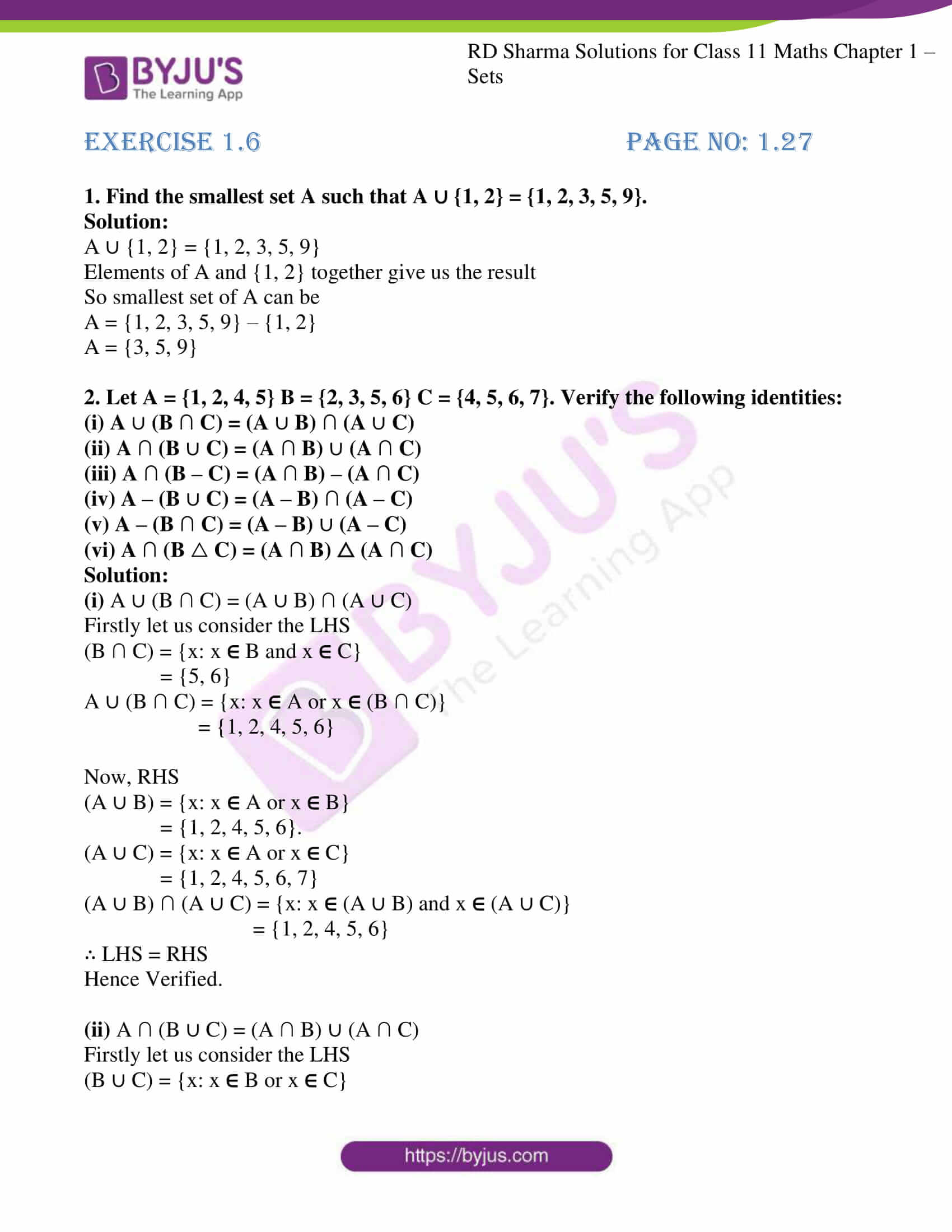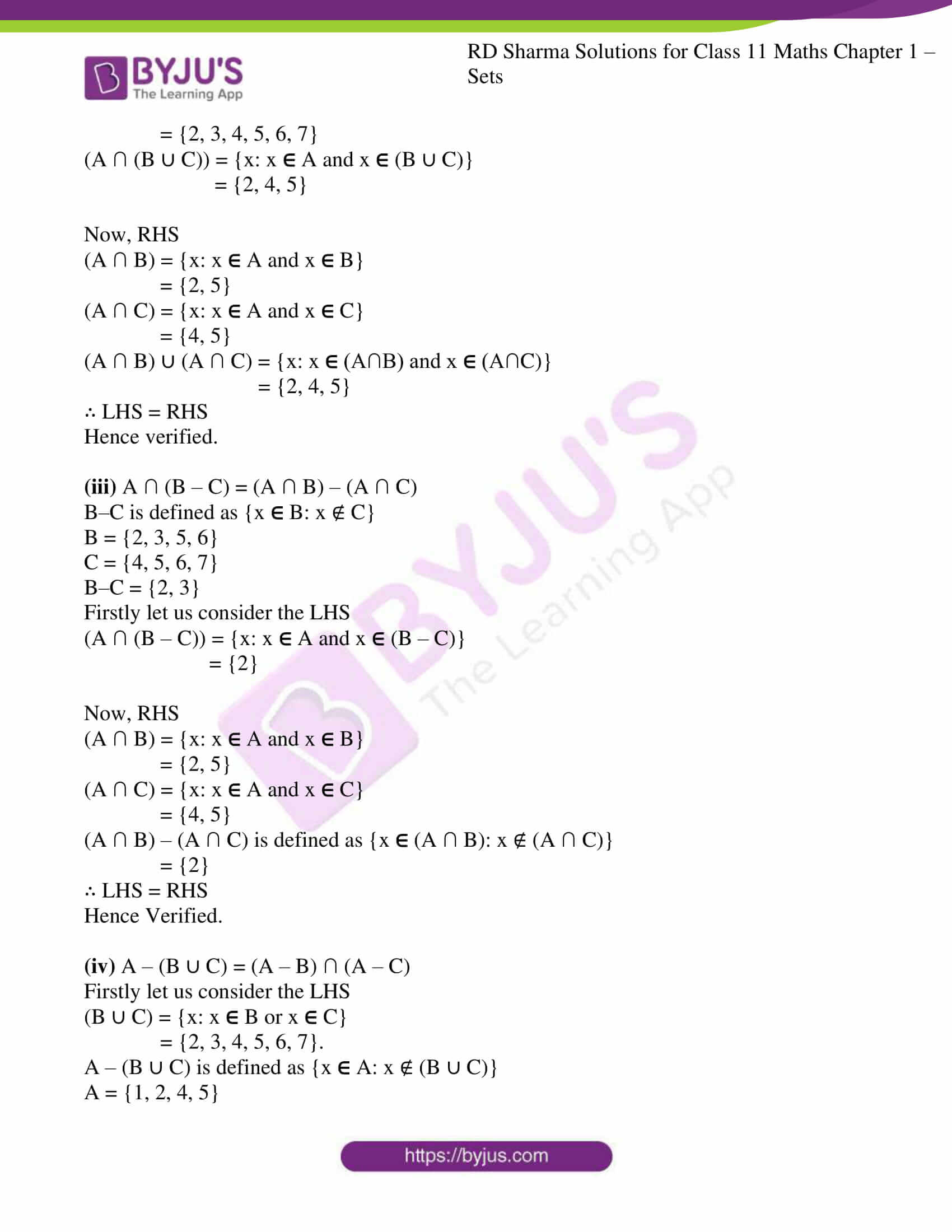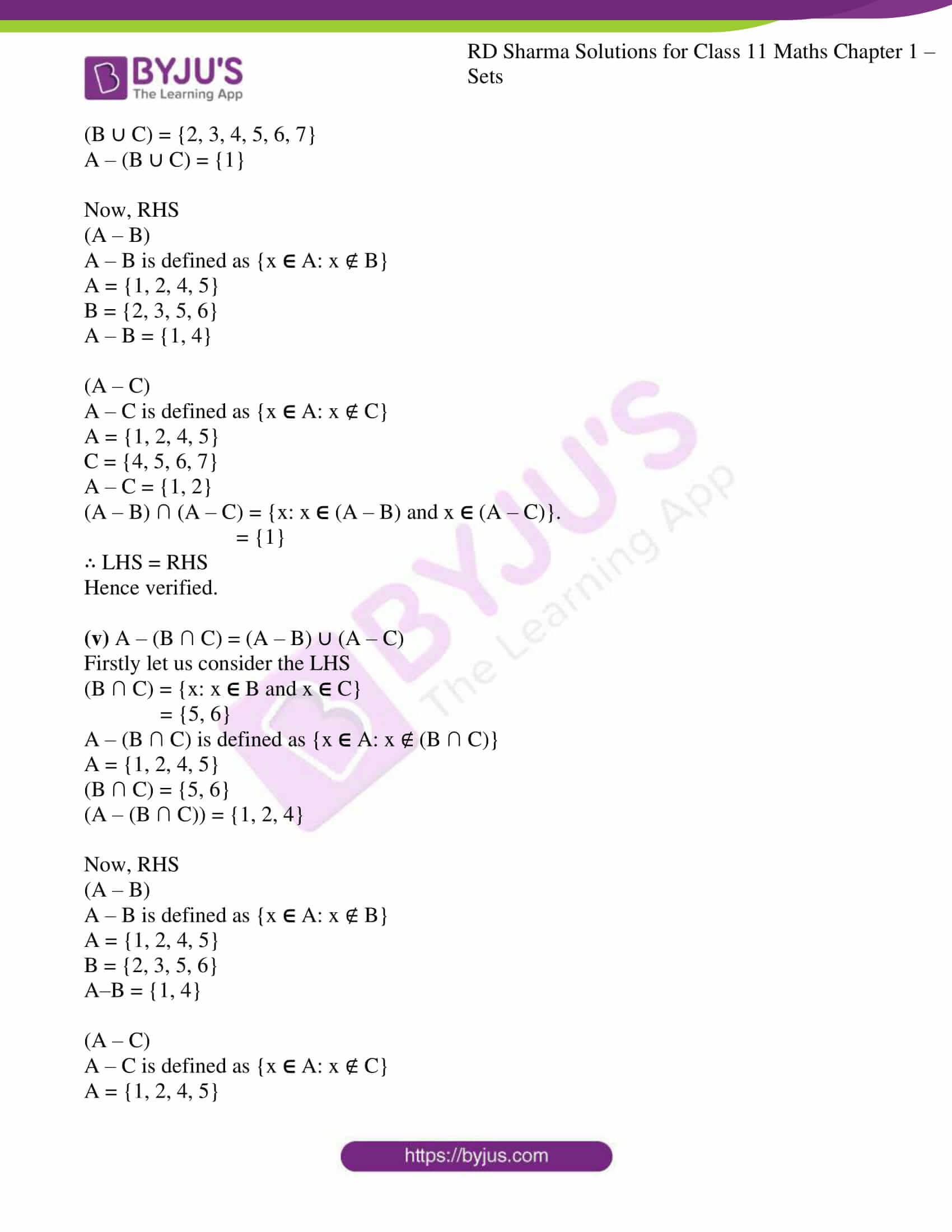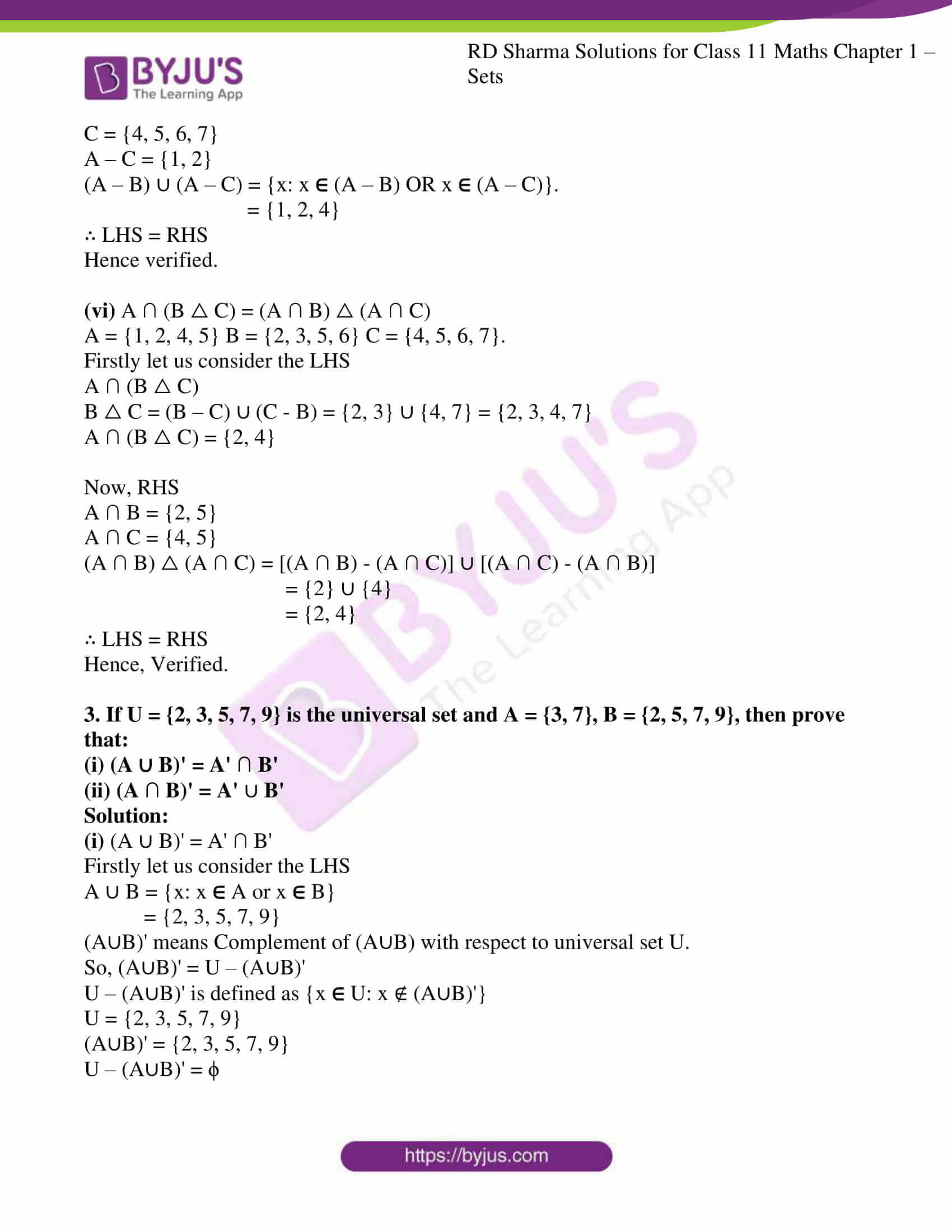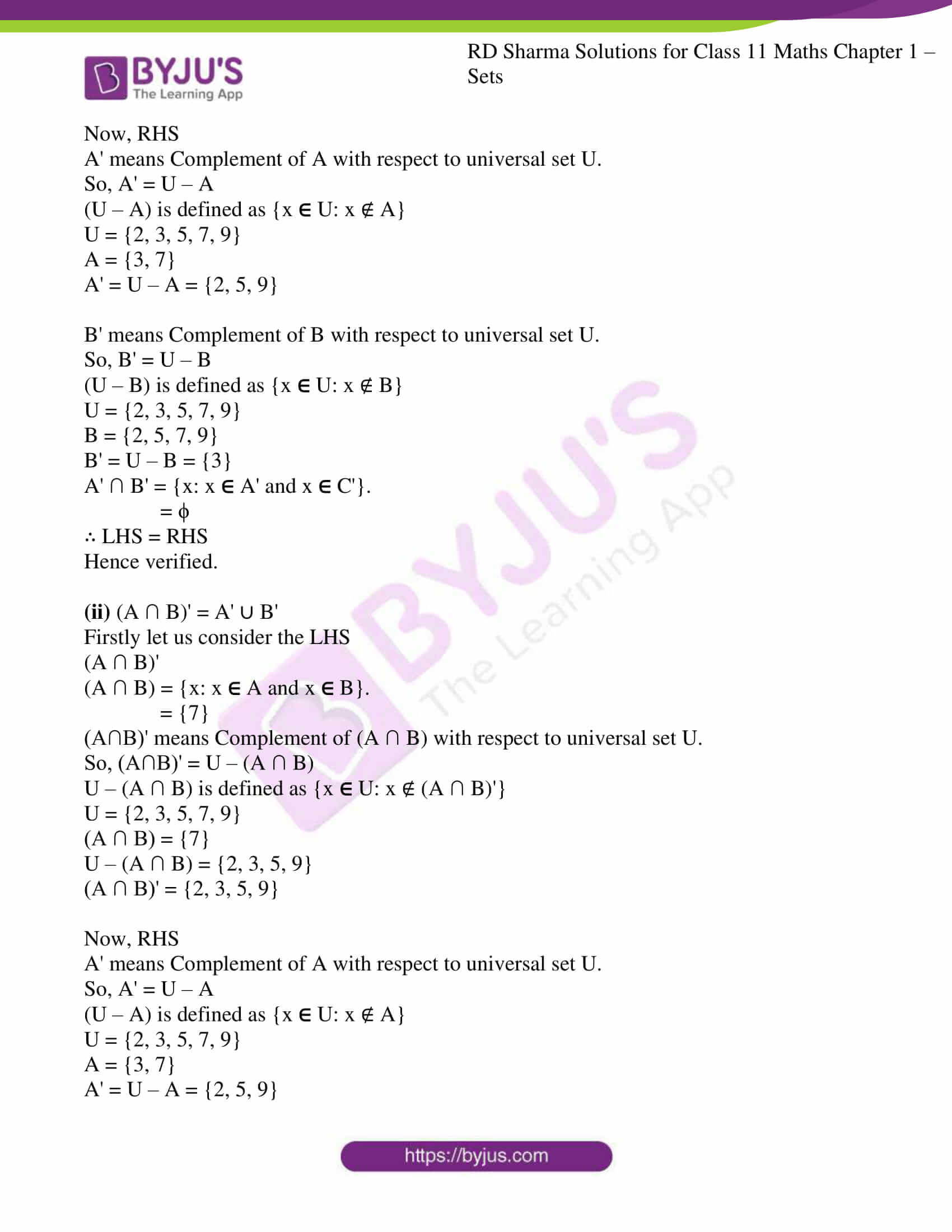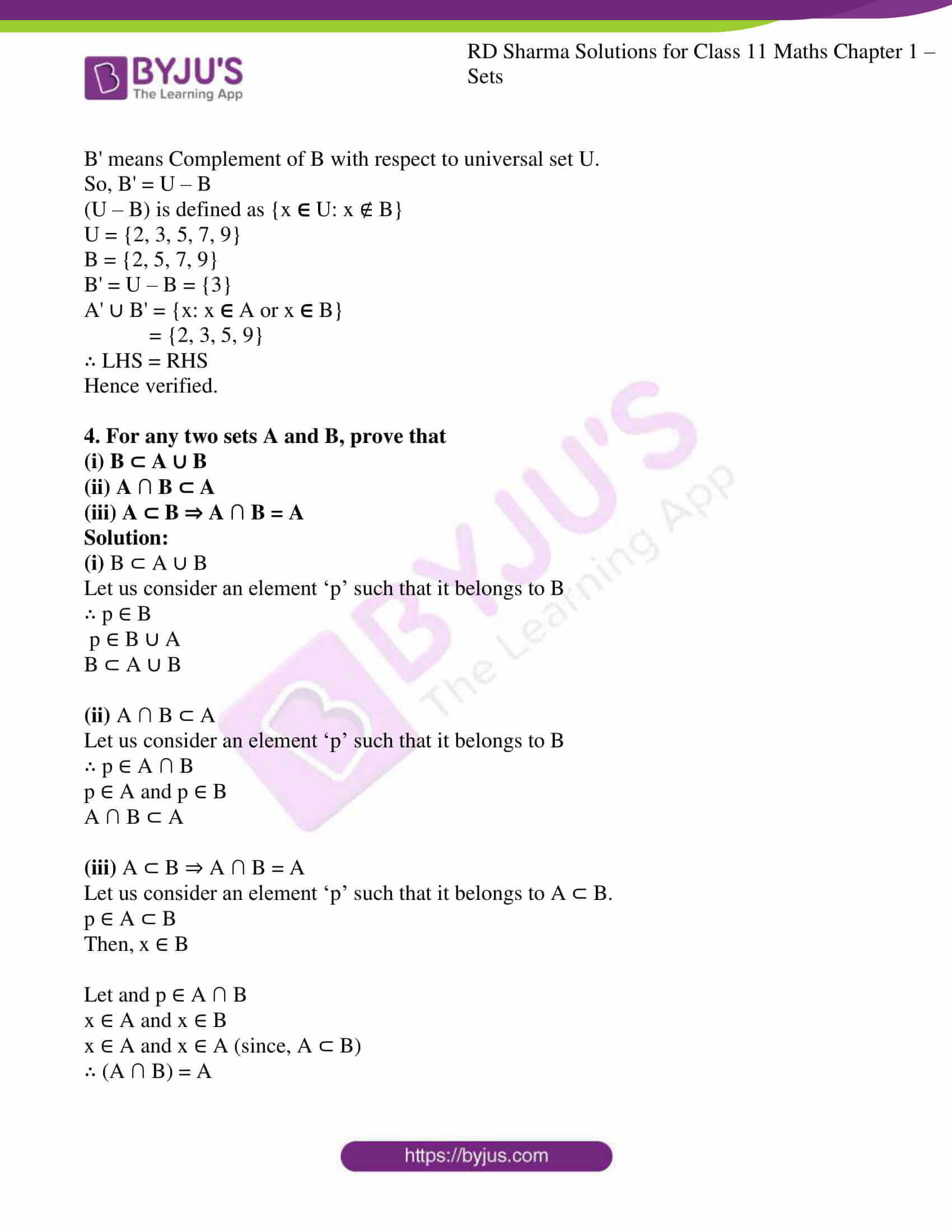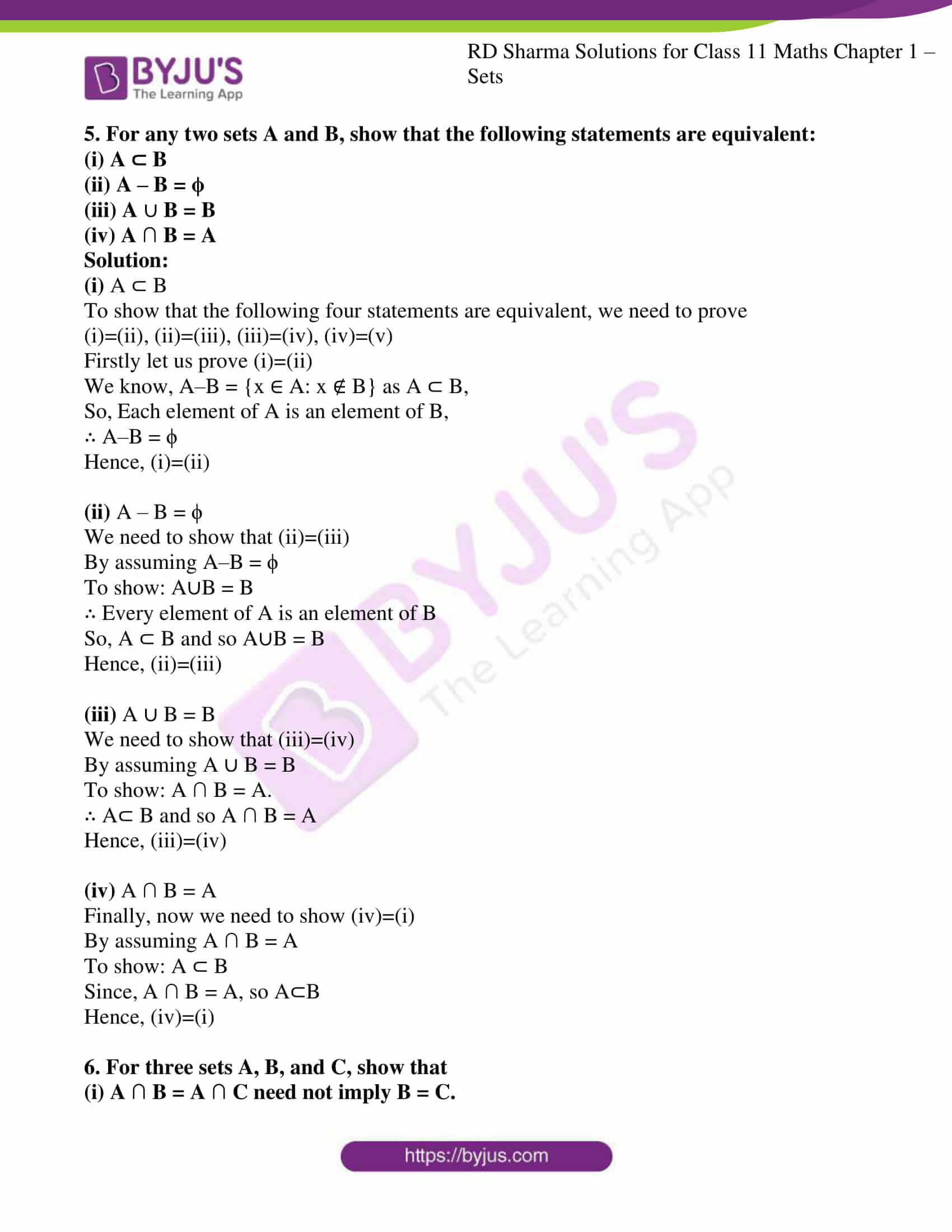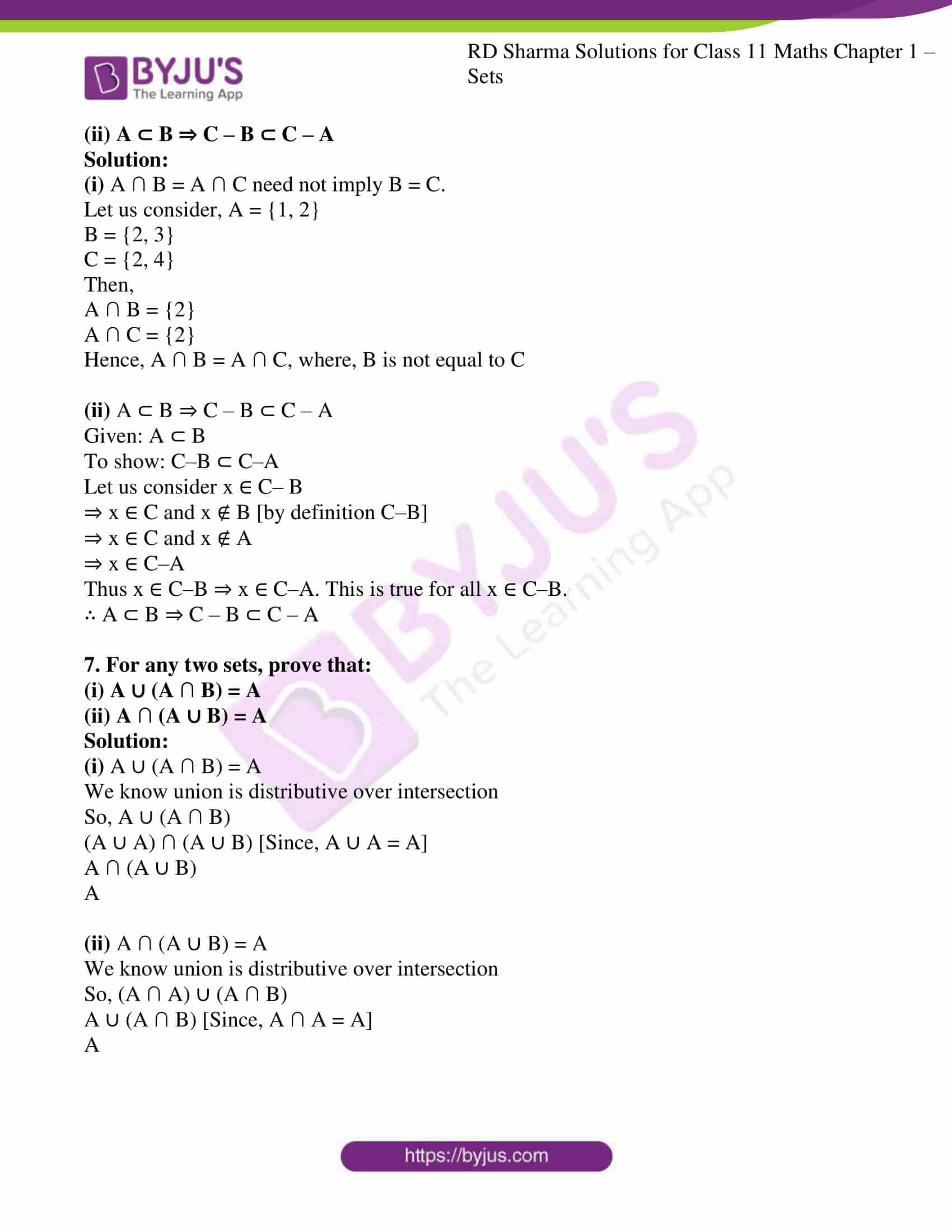### Access answers to RD Sharma Solutions for Class 11 Maths Exercise 1.6 Chapter 1 – Sets

1. Find the smallest set A such that A ∪ {1, 2} = {1, 2, 3, 5, 9}.

Solution:

A ∪ {1, 2} = {1, 2, 3, 5, 9}

Elements of A and {1, 2} together give us the result

So smallest set of A can be

A = {1, 2, 3, 5, 9} – {1, 2}

A = {3, 5, 9}

2. Let A = {1, 2, 4, 5} B = {2, 3, 5, 6} C = {4, 5, 6, 7}. Verify the following identities:
(i) A
(B ∩ C) = (A  B) ∩ (A  C)

(ii) A ∩ (B  C) = (A ∩ B)  (A ∩ C)

(iii) A ∩ (B – C) = (A ∩ B) – (A ∩ C)

(iv) A – (B ∪ C) = (A – B) ∩ (A – C)

(v) A – (B ∩ C) = (A – B)  (A – C)

(vi) A ∩ (B  C) = (A ∩ B) △ (A ∩ C)

Solution:

(i) A ∪ (B ∩ C) = (A ∪ B) ∩ (A ∪ C)

Firstly let us consider the LHS

(B ∩ C) = {x: x  B and x  C}

= {5, 6}

A ∪ (B ∩ C) = {x: x  A or x  (B ∩ C)}

= {1, 2, 4, 5, 6}

Now, RHS

(A ∪ B) = {x: x  A or x  B}

= {1, 2, 4, 5, 6}.

(A ∪ C) = {x: x  A or x  C}

= {1, 2, 4, 5, 6, 7}

(A ∪ B) ∩ (A ∪ C) = {x: x  (A ∪ B) and x  (A ∪ C)}

= {1, 2, 4, 5, 6}

∴ LHS = RHS

Hence Verified.

(ii) A ∩ (B ∪ C) = (A ∩ B) ∪ (A ∩ C)

Firstly let us consider the LHS

(B ∪ C) = {x: x  B or x  C}

= {2, 3, 4, 5, 6, 7}

(A ∩ (B ∪ C)) = {x: x  A and x  (B ∪ C)}

= {2, 4, 5}

Now, RHS

(A ∩ B) = {x: x  A and x  B}

= {2, 5}

(A ∩ C) = {x: x  A and x  C}

= {4, 5}

(A ∩ B) ∪ (A ∩ C) = {x: x  (A∩B) and x  (A∩C)}

= {2, 4, 5}

∴ LHS = RHS

Hence verified.

(iii) A ∩ (B – C) = (A ∩ B) – (A ∩ C)

B–C is defined as {x  B: x ∉ C}

B = {2, 3, 5, 6}

C = {4, 5, 6, 7}

B–C = {2, 3}

Firstly let us consider the LHS

(A ∩ (B – C)) = {x: x  A and x  (B – C)}

= {2}

Now, RHS

(A ∩ B) = {x: x  A and x  B}

= {2, 5}

(A ∩ C) = {x: x  A and x  C}

= {4, 5}

(A ∩ B) – (A ∩ C) is defined as {x  (A ∩ B): x ∉ (A ∩ C)}

= {2}

∴ LHS = RHS

Hence Verified.

(iv) A – (B ∪ C) = (A – B) ∩ (A – C)

Firstly let us consider the LHS

(B ∪ C) = {x: x  B or x  C}

= {2, 3, 4, 5, 6, 7}.

A – (B ∪ C) is defined as {x  A: x ∉ (B ∪ C)}

A = {1, 2, 4, 5}

(B ∪ C) = {2, 3, 4, 5, 6, 7}

A – (B ∪ C) = {1}

Now, RHS

(A – B)

A – B is defined as {x  A: x ∉ B}

A = {1, 2, 4, 5}

B = {2, 3, 5, 6}

A – B = {1, 4}

(A – C)

A – C is defined as {x  A: x ∉ C}

A = {1, 2, 4, 5}

C = {4, 5, 6, 7}

A – C = {1, 2}

(A – B) ∩ (A – C) = {x: x  (A – B) and x  (A – C)}.

= {1}

∴ LHS = RHS

Hence verified.

(v) A – (B ∩ C) = (A – B) ∪ (A – C)

Firstly let us consider the LHS

(B ∩ C) = {x: x  B and x  C}

= {5, 6}

A – (B ∩ C) is defined as {x  A: x ∉ (B ∩ C)}

A = {1, 2, 4, 5}

(B ∩ C) = {5, 6}

(A – (B ∩ C)) = {1, 2, 4}

Now, RHS

(A – B)

A – B is defined as {x  A: x ∉ B}

A = {1, 2, 4, 5}

B = {2, 3, 5, 6}

A–B = {1, 4}

(A – C)

A – C is defined as {x  A: x ∉ C}

A = {1, 2, 4, 5}

C = {4, 5, 6, 7}

A – C = {1, 2}

(A – B) ∪ (A – C) = {x: x  (A – B) OR x  (A – C)}.

= {1, 2, 4}

∴ LHS = RHS

Hence verified.

(vi) A ∩ (B △ C) = (A ∩ B) △ (A ∩ C)

A = {1, 2, 4, 5} B = {2, 3, 5, 6} C = {4, 5, 6, 7}.

Firstly let us consider the LHS

A ∩ (B △ C)

B △ C = (B – C) ∪ (C – B) = {2, 3} ∪ {4, 7} = {2, 3, 4, 7}

A ∩ (B △ C) = {2, 4}

Now, RHS

A ∩ B = {2, 5}

A ∩ C = {4, 5}

(A ∩ B) △ (A ∩ C) = [(A ∩ B) – (A ∩ C)] ∪ [(A ∩ C) – (A ∩ B)]

= {2} ∪ {4}

= {2, 4}

∴ LHS = RHS

Hence, Verified.

3. If U = {2, 3, 5, 7, 9} is the universal set and A = {3, 7}, B = {2, 5, 7, 9}, then prove that:
(i) (A ∪ B)’ = A’ ∩ B’

(ii) (A ∩ B)’ = A’  B’

Solution:

(i) (A ∪ B)’ = A’ ∩ B’

Firstly let us consider the LHS

A ∪ B = {x: x  A or x  B}

= {2, 3, 5, 7, 9}

(A∪B)’ means Complement of (A∪B) with respect to universal set U.

So, (A∪B)’ = U – (A∪B)’

U – (A∪B)’ is defined as {x  U: x ∉ (A∪B)’}

U = {2, 3, 5, 7, 9}

(A∪B)’ = {2, 3, 5, 7, 9}

U – (A∪B)’ = ϕ

Now, RHS

A’ means Complement of A with respect to universal set U.

So, A’ = U – A

(U – A) is defined as {x  U: x ∉ A}

U = {2, 3, 5, 7, 9}

A = {3, 7}

A’ = U – A = {2, 5, 9}

B’ means Complement of B with respect to universal set U.

So, B’ = U – B

(U – B) is defined as {x  U: x ∉ B}

U = {2, 3, 5, 7, 9}

B = {2, 5, 7, 9}

B’ = U – B = {3}

A’ ∩ B’ = {x: x  A’ and x  C’}.

= ϕ

∴ LHS = RHS

Hence verified.

(ii) (A ∩ B)’ = A’ ∪ B’

Firstly let us consider the LHS

(A ∩ B)’

(A ∩ B) = {x: x  A and x  B}.

= {7}

(A∩B)’ means Complement of (A ∩ B) with respect to universal set U.

So, (A∩B)’ = U – (A ∩ B)

U – (A ∩ B) is defined as {x  U: x ∉ (A ∩ B)’}

U = {2, 3, 5, 7, 9}

(A ∩ B) = {7}

U – (A ∩ B) = {2, 3, 5, 9}

(A ∩ B)’ = {2, 3, 5, 9}

Now, RHS

A’ means Complement of A with respect to universal set U.

So, A’ = U – A

(U – A) is defined as {x  U: x ∉ A}

U = {2, 3, 5, 7, 9}

A = {3, 7}

A’ = U – A = {2, 5, 9}

B’ means Complement of B with respect to universal set U.

So, B’ = U – B

(U – B) is defined as {x  U: x ∉ B}

U = {2, 3, 5, 7, 9}

B = {2, 5, 7, 9}

B’ = U – B = {3}

A’ ∪ B’ = {x: x  A or x  B}

= {2, 3, 5, 9}

∴ LHS = RHS

Hence verified.

4. For any two sets A and B, prove that
(i) B ⊂ A ∪ B

(ii) A ∩ B ⊂ A

(iii) A ⊂ B ⇒ A ∩ B = A

Solution:

(i) B ⊂ A ∪ B

Let us consider an element ‘p’ such that it belongs to B

∴ p ∈ B

p ∈ B ∪ A

B ⊂ A ∪ B

(ii) A ∩ B ⊂ A

Let us consider an element ‘p’ such that it belongs to B

∴ p ∈ A ∩ B

p ∈ A and p ∈ B

A ∩ B ⊂ A

(iii) A ⊂ B ⇒ A ∩ B = A

Let us consider an element ‘p’ such that it belongs to A ⊂ B.

p ∈ A ⊂ B

Then, x ∈ B

Let and p ∈ A ∩ B

x ∈ A and x ∈ B

x ∈ A and x ∈ A (since, A ⊂ B)

∴ (A ∩ B) = A

5. For any two sets A and B, show that the following statements are equivalent:
(i) A ⊂ B

(ii) A – B = ϕ

(iii) A  B = B

(iv) A ∩ B = A

Solution:

(i) A ⊂ B

To show that the following four statements are equivalent, we need to prove (i)=(ii), (ii)=(iii), (iii)=(iv), (iv)=(v)

Firstly let us prove (i)=(ii)

We know, A–B = {x ∈ A: x ∉ B} as A ⊂ B,

So, Each element of A is an element of B,

∴ A–B = ϕ

Hence, (i)=(ii)

(ii) A – B = ϕ

We need to show that (ii)=(iii)

By assuming A–B = ϕ

To show: A∪B = B

∴ Every element of A is an element of B

So, A ⊂ B and so A∪B = B

Hence, (ii)=(iii)

(iii) A ∪ B = B

We need to show that (iii)=(iv)

By assuming A ∪ B = B

To show: A ∩ B = A.

∴ A⊂ B and so A ∩ B = A

Hence, (iii)=(iv)

(iv) A ∩ B = A

Finally, now we need to show (iv)=(i)

By assuming A ∩ B = A

To show: A ⊂ B

Since, A ∩ B = A, so A⊂B

Hence, (iv)=(i)

6. For three sets A, B, and C, show that
(i) A ∩ B = A ∩ C need not imply B = C.

(ii) A ⊂ B ⇒ C – B ⊂ C – A

Solution:

(i) A ∩ B = A ∩ C need not imply B = C.

Let us consider, A = {1, 2}

B = {2, 3}

C = {2, 4}

Then,

A ∩ B = {2}

A ∩ C = {2}

Hence, A ∩ B = A ∩ C, where, B is not equal to C

(ii) A ⊂ B ⇒ C – B ⊂ C – A

Given: A ⊂ B

To show: C–B ⊂ C–A

Let us consider x ∈ C– B

⇒ x ∈ C and x ∉ B [by definition C–B]

⇒ x ∈ C and x ∉ A

⇒ x ∈ C–A

Thus x ∈ C–B ⇒ x ∈ C–A. This is true for all x ∈ C–B.

∴ A ⊂ B ⇒ C – B ⊂ C – A

7. For any two sets, prove that:
(i) A ∪ (A ∩ B) = A

(ii) A ∩ (A ∪ B) = A

Solution:

(i) A ∪ (A ∩ B) = A

We know union is distributive over intersection

So, A ∪ (A ∩ B)

(A ∪ A) ∩ (A ∪ B) [Since, A ∪ A = A]

A ∩ (A ∪ B)

A

(ii) A ∩ (A ∪ B) = A

We know union is distributive over intersection

So, (A ∩ A) ∪ (A ∩ B)

A ∪ (A ∩ B) [Since, A ∩ A = A]

A